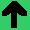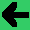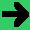# Lab 13: Eight Queens

1. The task today is to search for a way to place eight queens on a chess board so that none can take each other.
2. Open a project and make a hello world.
3. You can do the rest of this lab one of two ways, start with the four queens problem, or start with the eight queens problem. The directions here will go from the four.
4. Make an 4x4 array of booleans, all initially set to false.
5. Make a function to see if a queen can take another queen; the inputs to the function are the x and y coordinates of the two queens. (They can take each other if they're on the same row, the same column, or diagonal (either way) to each other.)
6. Now, place the first queen (the one in the 0 column) in the first (0) row. Then search for the place the second queen can go.
7. Add a loop for the third queen.
8. Add a loop for the first, and fourth queen. This should be four nested loops.
9. You should be able to print out all of the ways the four queens can be set.
10. Now, make the board 8x8.
11. Now add the four other nested loops.
1. If you get this done, try the whole task again using the constraint satisfaction algebra. Indeed, if you're bold, you can start with this.